Quantity: 0

Total: 0,00

0

# p-V-T diagram for ideal gases

### p-V-T diagram for ideal gases

The relationship between the pressure, volume and temperature of ideal gases is described by the gas laws.

Physics

Keywords

p-V-T diagram, ideal gas, gas laws, Boyle-Mariotte law, Gay-Lussac´s 1st law, Gay-Lussac´s 2nd law, P-v-T surface, isochoric process, isothermal process, isobaric process, value of a quantity, state quantity, thermodynamics, temperature, pressure, volume, gas, temperature change, volumetric thermal expansion, physical property, physics, physical

Related items

### Scenes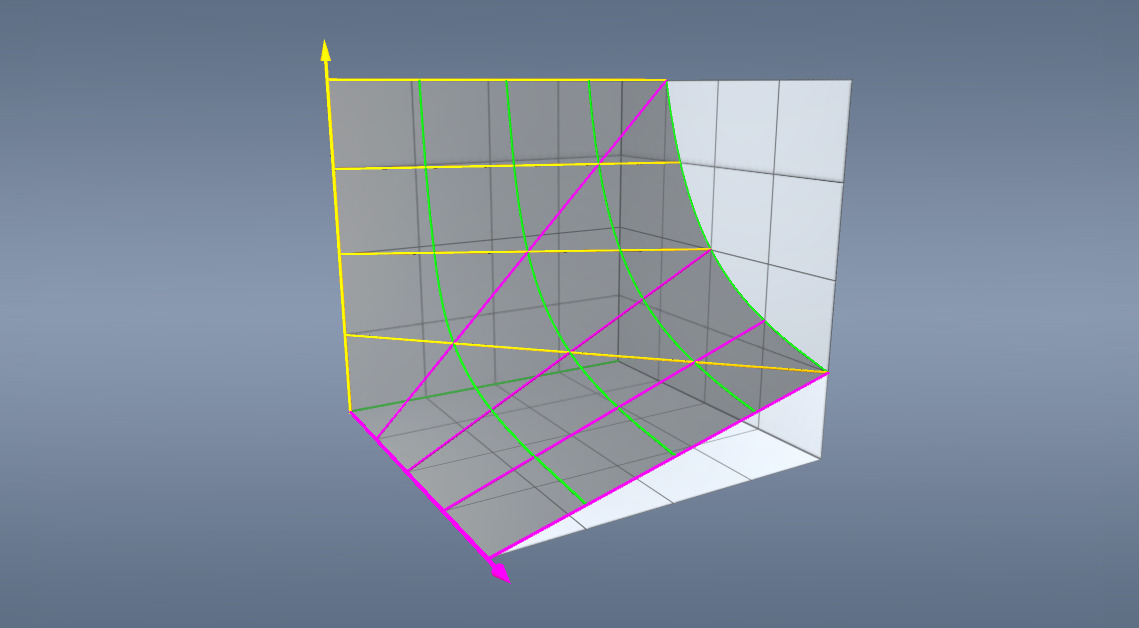### p-V-T diagram

• p - Pressure
• V - Volume
• T - Temperature
• p ₁
• p ₂
• p ₃
• p ₄
• T ₁
• T ₂
• T ₃
• T ₄
• V ₁
• V ₂
• V ₃
• V ₄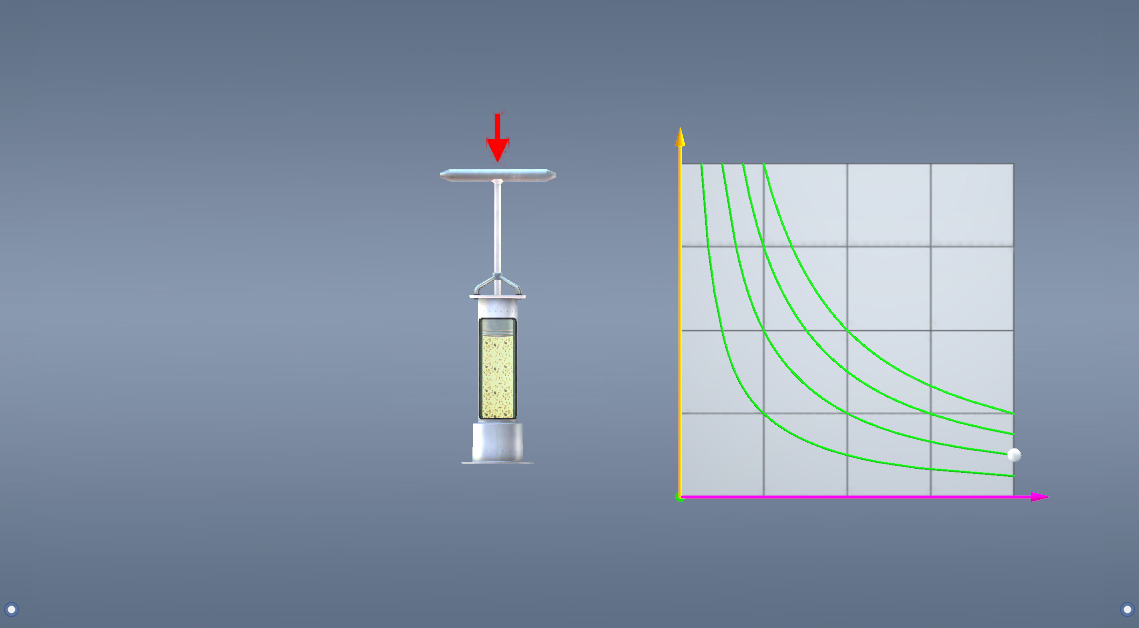### Constant temperature

• p - Pressure
• V - Volume
• T = constant

When we view the p-V-T diagram from the T axis, we see a two-dimensional p-V diagram which describes corresponding changes in the volume and pressure of the gas at a fixed temperature.

When the gas is compressed at a constant temperature, its pressure increases. To ensure this, the gas has to release heat while being compressed and absorb heat while expanding.

This change is described by the Boyle-Mariotte law: if the temperature remains constant, the pressure and volume of a given amount of gas are inversely proportional.

The process takes place along isotherms.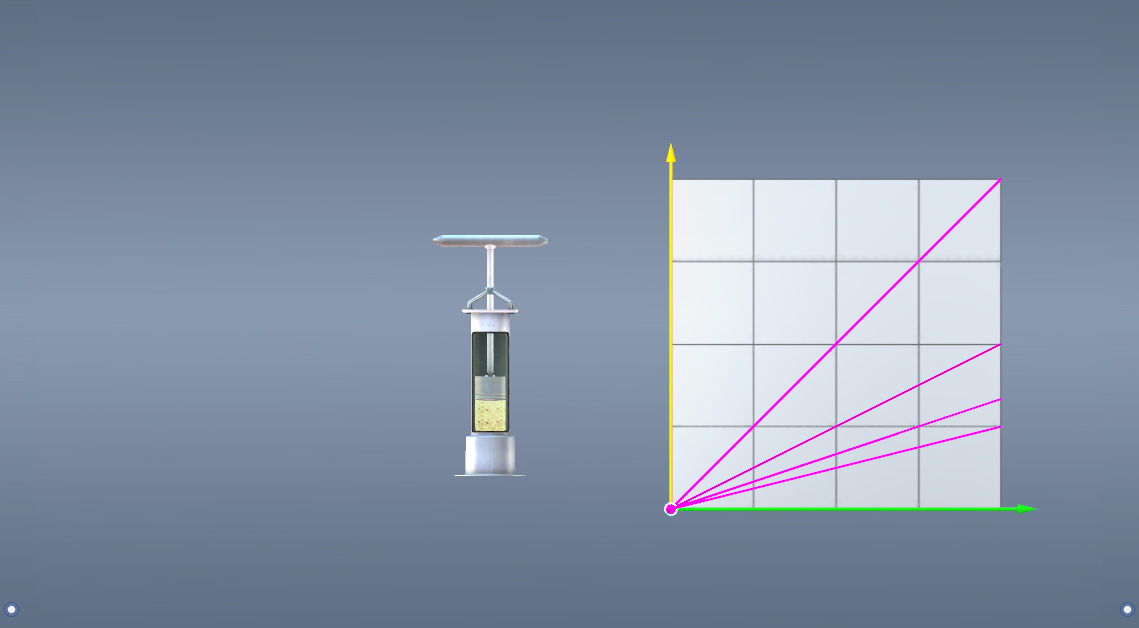### Constant volume

• p - Pressure
• V = constant
• T - Temperature

When we view the p-V-T diagram from the V axis, we see a two-dimensional p-T diagram which describes corresponding changes in the pressure and temperature of the gas at a fixed volume.

When the gas is heated while its volume is unchanged, its pressure will increase. This change is described by Gay-Lussac´s 2nd law: if the volume remains constant, the pressure of a given amount of gas is in direct proportion to its temperature. The process takes place along isochors.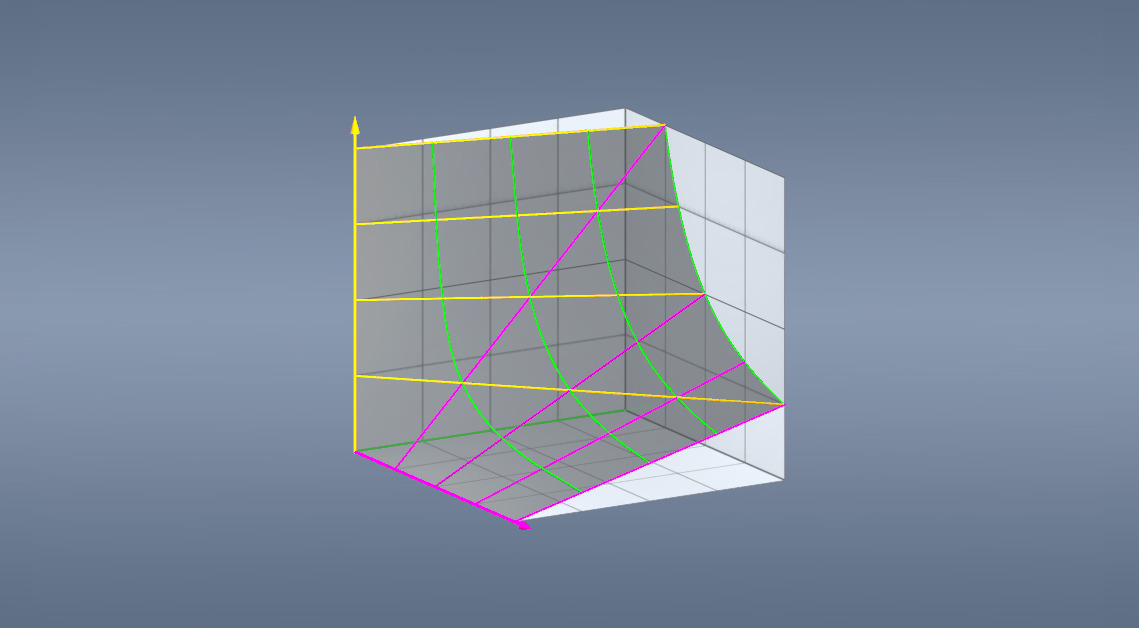### Constant pressure

• p = constant
• V - Volume
• T - Temperature

When we view the p-V-T diagram from the p axis, we see a two-dimensional V-T diagram which describes corresponding changes in the temperature and volume of the gas at a fixed pressure.

When the gas is heated while the pressure remains unchanged, its volume will increase. This change is described by Gay-Lussac´s 1st law: if the pressure remains constant, the volume and temperature of a given amount of gas are directly proportional. The process takes place along isobars.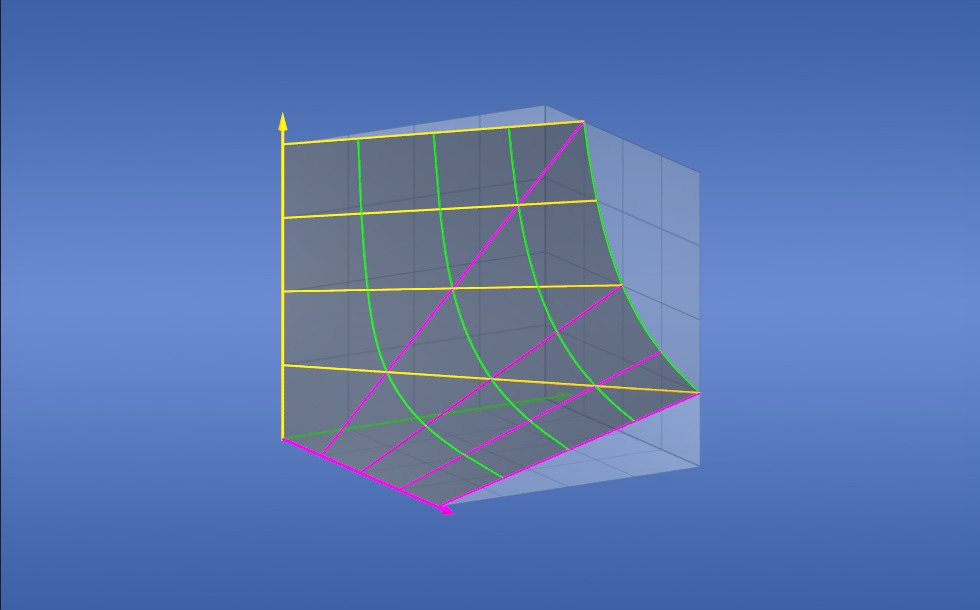### p-V-T surface

Correlations between the pressure, volume and temperature of a given amount of ideal gas may be described in three-dimensional graphs showing the three thermodynamic quantities.
The possible equilibrium conditions determine a 3D surface. Ideal gases occur in states that are on this surface. Therefore, if the values of two of the quantities are known, the value of the third can be determined, for example the volume of the gas can be calculated if the temperature and pressure are known.

### Related items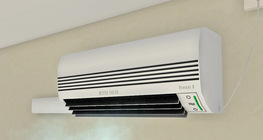#### How does it work? - Air conditioner

An air conditioner cools the air inside by drawing heat away and releasing it outside.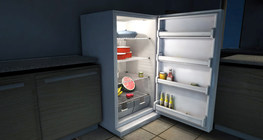#### How does it work - Refrigerator

This animation demonstrates how a refrigerator works.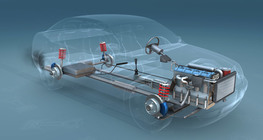#### Diesel engine

The German engineer Rudolf Diesel patented the diesel engine in 1893.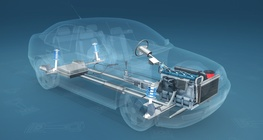#### Four-stroke Otto engine

This animation demonstrates the type of engine most commonly used in cars.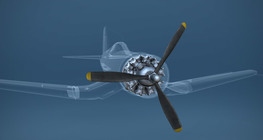Radial engines are used primarily in aeroplanes and helicopters.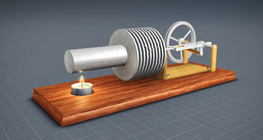#### Stirling engine - hot-air engine

Stirling engines are also known as external combustion engines. Unlike internal combustion engines (e.g. Otto-engine), here combustion takes place outside...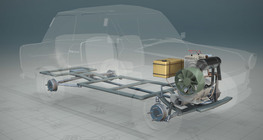#### Two-stroke engine

A two-stroke engine is a type of internal combustion engine with a cycle of only two (power) strokes.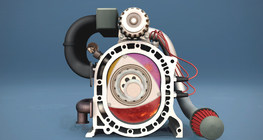#### Wankel engine

A type of rotary engine of high efficiency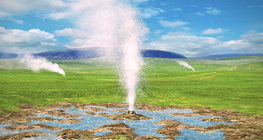#### Geyser

A geyser is a spring characterised by intermittent discharge of water and steam.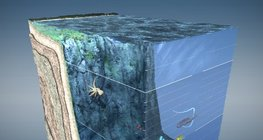#### Layers of the ocean

Physical properties, as well as the flora and fauna of the ocean change with depth.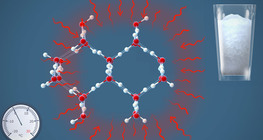#### Melting and freezing

During freezing hydrogen bonds are formed between water molecules resulting in a crystal structure.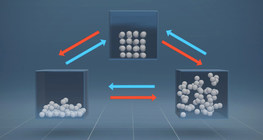#### Phase transitions

A phase transition is the change of a substance from one state of matter to another.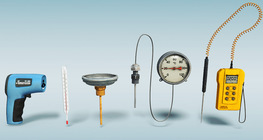#### Thermometers

There are various types of instruments used for measuring temperature.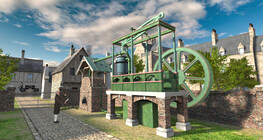#### Watt´s steam engine (18th century)

The steam engine, perfected by the Scottish engineer James Watt, revolutionised technology.#### Body-centred cubic lattice

The least tightly packed crystal structure.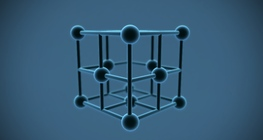#### Face-centred cubic metal lattice

The face-centred cubic metal lattice allows the closest fit of metal atoms.#### Hexagonal metallic lattice

Metals forming hexagonal metallic lattices are rigid and difficult to machine.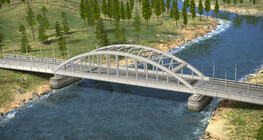#### Thermal expansion of bridges

The length of the metal frameworks of bridges change when the temperature changes.